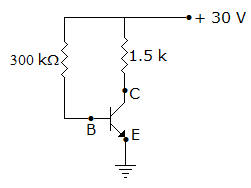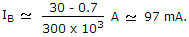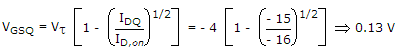# Electronics and Communication Engineering - Analog Electronics

46.

A transistor has a power rating of 8 W for a case temperature of 25°C. If derating factor is 30 mW/°C, the power rating for 55°C, case temperature is

 A. 8 W B. 7.5 W C. 7.1 W D. 6.8 W

Explanation:

Power rating = 8 - (55 - 25)(30 x 10-3) = 7.1 W.

47.

In figure VBE = 0.7 V. The base current isExplanation:48.

An ideal power supply has

 A. zero internal resistance B. very high internal resistance C. high output resistance D. both (b) and (c)

Explanation:

An ideal power supply should have zero voltage drop.

49.

In a self bias circuit for CE amplifier, the emitter resistance is made three times the original value. The collector current will

 A. remain the same B. become three times C. decrease D. become one-third

Explanation:

The emitter current becomes one-third since collector current in BJT is almost the same as emitter current, collector current also becomes one-third.

50.

A P-channel MOSFET operating in the enhancement mode is characterized by Vt = - 4 V and IDQ = - 10 mA, when VGSQ = - 5.5 V, what will be VGSQ if IDQ = -15 mA and ID, on = - 16 mA

 A. - 2 V B. 3 V C. 0.13 V D. 1.3 V.